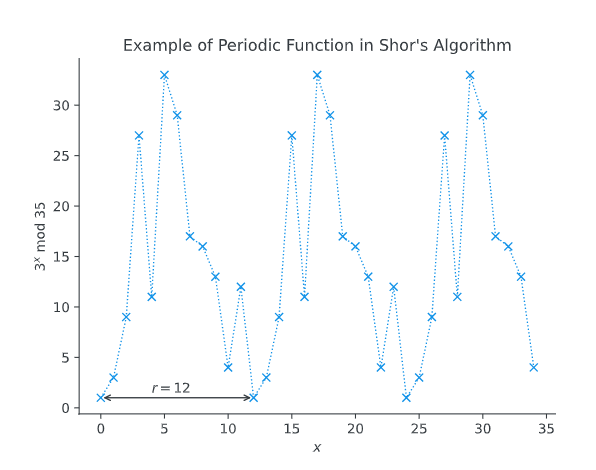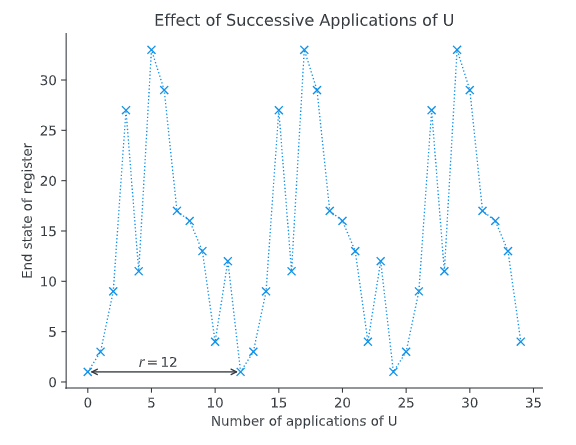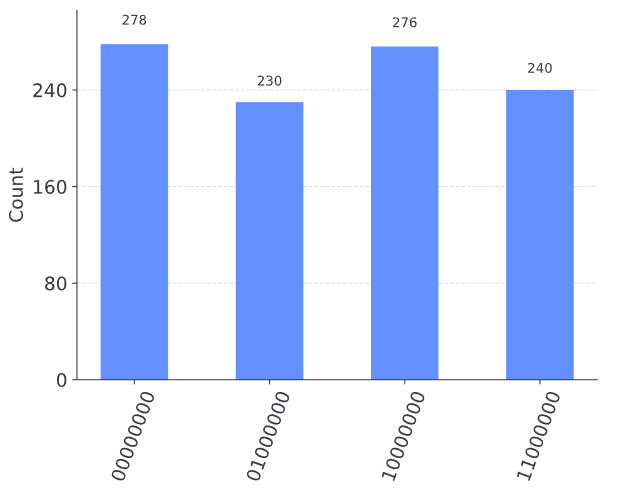# Shor算法

Shor算法因在多项式时间内分解整数而闻名。由于最著名的经典算法需要超多项式时间来分解两个素数的乘积，因此广泛使用的密码系统RSA依赖于因子分解对于足够大的整数是不可能的。

import matplotlib.pyplot as plt
import numpy as np
from qiskit import QuantumCircuit, Aer, transpile, assemble
from qiskit.visualization import plot_histogram
from math import gcd
from numpy.random import randint
import pandas as pd
from fractions import Fraction
print("Imports Successful")


## 1. 问题在于:周期查找

f(x) = a^x \bmod{N}

17 \bmod 5 = 2

17 = 2 \pmod 5

a^r \bmod N = 1## 2.解决方案

Shor的解决方案是在酉算子上使用量子相位估计:

U|y\rangle \equiv |ay \bmod N \rangle

\begin{aligned} U|1\rangle &= |3\rangle & \\ U^2|1\rangle &= |9\rangle \\ U^3|1\rangle &= |27\rangle \\ & \vdots \\ U^{(r-1)}|1\rangle &= |12\rangle \\ U^r|1\rangle &= |1\rangle \end{aligned}|u_0\rangle = \tfrac{1}{\sqrt{r}}\sum_{k=0}^{r-1}{|a^k \bmod N\rangle}

\begin{aligned} |u_0\rangle &= \tfrac{1}{\sqrt{12}}(|1\rangle + |3\rangle + |9\rangle \dots + |4\rangle + |12\rangle) \\[10pt] U|u_0\rangle &= \tfrac{1}{\sqrt{12}}(U|1\rangle + U|3\rangle + U|9\rangle \dots + U|4\rangle + U|12\rangle) \\[10pt] &= \tfrac{1}{\sqrt{12}}(|3\rangle + |9\rangle + |27\rangle \dots + |12\rangle + |1\rangle) \\[10pt] &= |u_0\rangle \end{aligned}

\begin{aligned} |u_1\rangle &= \tfrac{1}{\sqrt{r}}\sum_{k=0}^{r-1}{e^{-\tfrac{2\pi i k}{r}}|a^k \bmod N\rangle}\\[10pt] U|u_1\rangle &= e^{\tfrac{2\pi i}{r}}|u_1\rangle \end{aligned}

\begin{aligned} |u_1\rangle &= \tfrac{1}{\sqrt{12}}(|1\rangle + e^{-\tfrac{2\pi i}{12}}|3\rangle + e^{-\tfrac{4\pi i}{12}}|9\rangle \dots + e^{-\tfrac{20\pi i}{12}}|4\rangle + e^{-\tfrac{22\pi i}{12}}|12\rangle) \\[10pt] U|u_1\rangle &= \tfrac{1}{\sqrt{12}}(|3\rangle + e^{-\tfrac{2\pi i}{12}}|9\rangle + e^{-\tfrac{4\pi i}{12}}|27\rangle \dots + e^{-\tfrac{20\pi i}{12}}|12\rangle + e^{-\tfrac{22\pi i}{12}}|1\rangle) \\[10pt] U|u_1\rangle &= e^{\tfrac{2\pi i}{12}}\cdot\tfrac{1}{\sqrt{12}}(e^{\tfrac{-2\pi i}{12}}|3\rangle + e^{-\tfrac{4\pi i}{12}}|9\rangle + e^{-\tfrac{6\pi i}{12}}|27\rangle \dots + e^{-\tfrac{22\pi i}{12}}|12\rangle + e^{-\tfrac{24\pi i}{12}}|1\rangle) \\[10pt] U|u_1\rangle &= e^{\tfrac{2\pi i}{12}}|u_1\rangle \end{aligned}

\begin{aligned} |u_s\rangle &= \tfrac{1}{\sqrt{r}}\sum_{k=0}^{r-1}{e^{-\tfrac{2\pi i s k}{r}}|a^k \bmod N\rangle}\\[10pt] U|u_s\rangle &= e^{\tfrac{2\pi i s}{r}}|u_s\rangle \end{aligned}

\begin{aligned} |u_s\rangle &= \tfrac{1}{\sqrt{12}}(|1\rangle + e^{-\tfrac{2\pi i s}{12}}|3\rangle + e^{-\tfrac{4\pi i s}{12}}|9\rangle \dots + e^{-\tfrac{20\pi i s}{12}}|4\rangle + e^{-\tfrac{22\pi i s}{12}}|12\rangle) \\[10pt] U|u_s\rangle &= \tfrac{1}{\sqrt{12}}(|3\rangle + e^{-\tfrac{2\pi i s}{12}}|9\rangle + e^{-\tfrac{4\pi i s}{12}}|27\rangle \dots + e^{-\tfrac{20\pi i s}{12}}|12\rangle + e^{-\tfrac{22\pi i s}{12}}|1\rangle) \\[10pt] U|u_s\rangle &= e^{\tfrac{2\pi i s}{12}}\cdot\tfrac{1}{\sqrt{12}}(e^{-\tfrac{2\pi i s}{12}}|3\rangle + e^{-\tfrac{4\pi i s}{12}}|9\rangle + e^{-\tfrac{6\pi i s}{12}}|27\rangle \dots + e^{-\tfrac{22\pi i s}{12}}|12\rangle + e^{-\tfrac{24\pi i s}{12}}|1\rangle) \\[10pt] U|u_s\rangle &= e^{\tfrac{2\pi i s}{12}}|u_s\rangle \end{aligned}

0 \leq s \leq r-1.

\tfrac{1}{\sqrt{r}}\sum_{s=0}^{r-1} |u_s\rangle = |1\rangle

\begin{aligned} \tfrac{1}{2}(\quad|u_0\rangle &= \tfrac{1}{2}(|1\rangle \hphantom{e^{-\tfrac{2\pi i}{12}}}+ |7\rangle \hphantom{e^{-\tfrac{12\pi i}{12}}} + |4\rangle \hphantom{e^{-\tfrac{12\pi i}{12}}} + |13\rangle)\dots \\[10pt] + |u_1\rangle &= \tfrac{1}{2}(|1\rangle + e^{-\tfrac{2\pi i}{4}}|7\rangle + e^{-\tfrac{\hphantom{1}4\pi i}{4}}|4\rangle + e^{-\tfrac{\hphantom{1}6\pi i}{4}}|13\rangle)\dots \\[10pt] + |u_2\rangle &= \tfrac{1}{2}(|1\rangle + e^{-\tfrac{4\pi i}{4}}|7\rangle + e^{-\tfrac{\hphantom{1}8\pi i}{4}}|4\rangle + e^{-\tfrac{12\pi i}{4}}|13\rangle)\dots \\[10pt] + |u_3\rangle &= \tfrac{1}{2}(|1\rangle + e^{-\tfrac{6\pi i}{4}}|7\rangle + e^{-\tfrac{12\pi i}{4}}|4\rangle + e^{-\tfrac{18\pi i}{4}}|13\rangle)\quad) = |1\rangle \\[10pt] \end{aligned}

\phi = \frac{s}{r}## 3.使用Qiskit实现

U|y\rangle = |ay\bmod 15\rangle

def c_amod15(a, power):
"""Controlled multiplication by a mod 15"""
if a not in [2,4,7,8,11,13]:
raise ValueError("'a' must be 2,4,7,8,11 or 13")
U = QuantumCircuit(4)
for iteration in range(power):
if a in [2,13]:
U.swap(2,3)
U.swap(1,2)
U.swap(0,1)
if a in [7,8]:
U.swap(0,1)
U.swap(1,2)
U.swap(2,3)
if a in [4, 11]:
U.swap(1,3)
U.swap(0,2)
if a in [7,11,13]:
for q in range(4):
U.x(q)
U = U.to_gate()
U.name = "%i^%i mod 15" % (a, power)
c_U = U.control()
return c_U


# Specify variables
n_count = 8  # number of counting qubits
a = 7


def qft_dagger(n):
"""n-qubit QFTdagger the first n qubits in circ"""
qc = QuantumCircuit(n)
# Don't forget the Swaps!
for qubit in range(n//2):
qc.swap(qubit, n-qubit-1)
for j in range(n):
for m in range(j):
qc.cp(-np.pi/float(2**(j-m)), m, j)
qc.h(j)
qc.name = "QFT†"
return qc


# Create QuantumCircuit with n_count counting qubits
# plus 4 qubits for U to act on
qc = QuantumCircuit(n_count + 4, n_count)

# Initialize counting qubits
# in state |+>
for q in range(n_count):
qc.h(q)

# And auxiliary register in state |1>
qc.x(n_count)

# Do controlled-U operations
for q in range(n_count):
qc.append(c_amod15(a, 2**q),
[q] + [i+n_count for i in range(4)])

# Do inverse-QFT
qc.append(qft_dagger(n_count), range(n_count))

# Measure circuit
qc.measure(range(n_count), range(n_count))
qc.draw(fold=-1)  # -1 means 'do not fold'


aer_sim = Aer.get_backend('aer_simulator')
t_qc = transpile(qc, aer_sim)
qobj = assemble(t_qc)
results = aer_sim.run(qobj).result()
counts = results.get_counts()
plot_histogram(counts)rows, measured_phases = [], []
for output in counts:
decimal = int(output, 2)  # Convert (base 2) string to decimal
phase = decimal/(2**n_count)  # Find corresponding eigenvalue
measured_phases.append(phase)
# Add these values to the rows in our table:
rows.append([f"{output}(bin) = {decimal:>3}(dec)",
f"{decimal}/{2**n_count} = {phase:.2f}"])
# Print the rows in a table
print(df)


Fraction(0.666)


# Get fraction that most closely resembles 0.666
# with denominator < 15
Fraction(0.666).limit_denominator(15)


rows = []
for phase in measured_phases:
frac = Fraction(phase).limit_denominator(15)
rows.append([phase, f"{frac.numerator}/{frac.denominator}", frac.denominator])
# Print as a table
print(df)


• 修改上面的电路，使 $a$的值为 2,8,1113 。你得到了什么结果?为什么?

## 4.模幂运算

U^{2^j}|y\rangle = |a^{2^j}y \bmod N \rangle

a^{2^j} \bmod N

def a2jmodN(a, j, N):
"""Compute a^{2^j} (mod N) by repeated squaring"""
for i in range(j):
a = np.mod(a**2, N)
return a

a2jmodN(7, 2049, 53)


## 5.从周期查找中提取因子

Example:Factoring 15

N=15


np.random.seed(1) # This is to make sure we get reproduceable results
a = randint(2, 15)
print(a)


from math import gcd # greatest common divisor
gcd(a, N)


a^r \bmod N = 1

def qpe_amod15(a):
n_count = 8
qc = QuantumCircuit(4+n_count, n_count)
for q in range(n_count):
qc.h(q)     # Initialize counting qubits in state |+>
qc.x(3+n_count) # And auxiliary register in state |1>
for q in range(n_count): # Do controlled-U operations
qc.append(c_amod15(a, 2**q),
[q] + [i+n_count for i in range(4)])
qc.append(qft_dagger(n_count), range(n_count)) # Do inverse-QFT
qc.measure(range(n_count), range(n_count))
# Simulate Results
aer_sim = Aer.get_backend('aer_simulator')
# Setting memory=True below allows us to see a list of each sequential reading
t_qc = transpile(qc, aer_sim)
qobj = assemble(t_qc, shots=1)
result = aer_sim.run(qobj, memory=True).result()
print("Corresponding Phase: %f" % phase)
return phase


phase = qpe_amod15(a) # Phase = s/r
Fraction(phase).limit_denominator(15) # Denominator should (hopefully!) tell us r

frac = Fraction(phase).limit_denominator(15)
s, r = frac.numerator, frac.denominator
print(r)


a^r \bmod N = 1

(a^r - 1) \bmod N = 0

a^r -1 = (a^{r/2}-1)(a^{r/2}+1)

(如果 r 不是偶数，我们不能进一步，必须对 a 使用不同的值再次尝试)。那么 Na^{r/2}-1a^{r/2}+1 的最大公约数很可能是 N 的真因子:

guesses = [gcd(a**(r//2)-1, N), gcd(a**(r//2)+1, N)]
print(guesses)


a = 7
factor_found = False
attempt = 0
while not factor_found:
attempt += 1
print("\nAttempt %i:" % attempt)
phase = qpe_amod15(a) # Phase = s/r
frac = Fraction(phase).limit_denominator(N) # Denominator should (hopefully!) tell us r
r = frac.denominator
print("Result: r = %i" % r)
if phase != 0:
# Guesses for factors are gcd(x^{r/2} ±1 , 15)
guesses = [gcd(a**(r//2)-1, N), gcd(a**(r//2)+1, N)]
print("Guessed Factors: %i and %i" % (guesses, guesses))
for guess in guesses:
if guess not in [1,N] and (N % guess) == 0: # Check to see if guess is a factor
print("*** Non-trivial factor found: %i ***" % guess)
factor_found = True


## 6.参考文献

1. Stephane Beauregard, Circuit for Shor’s algorithm using 2n+3 qubits, arXiv:quant-ph/0205095

2. M. Nielsen and I. Chuang, Quantum Computation and Quantum Information, Cambridge Series on Information and the Natural Sciences (Cambridge University Press, Cambridge, 2000). (Page 633)

import qiskit.tools.jupyter
%qiskit_version_table


### 版本信息

Qiskit Software Version
qiskit-terra 0.22.0
qiskit-aer 0.11.0
qiskit-ibmq-provider 0.19.2
qiskit 0.39.0
qiskit-nature 0.4.1
qiskit-finance 0.3.2
qiskit-optimization 0.4.0
qiskit-machine-learning 0.4.0
System information
Python version 3.8.13
Python compiler Clang 13.1.6 (clang-1316.0.21.2.5)
Python build default, Aug 29 2022 05:17:23
OS Darwin
CPUs 8
Memory (Gb) 32.0
Thu Nov 24 11:55:19 2022 GMT